Next: Upwind schemes Up: Non-oscillatory central schemes Previous: Introduction

# A Short Guide to Godunov-Type schemes

We want to solve the hyperbolic system of conservation laws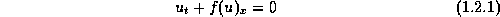by Godunov-type schemes. To this end we proceed in two steps. First, we introduce a small spatial scale,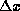, and we consider the corresponding (Steklov) sliding average of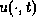,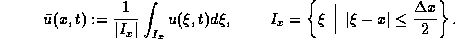The sliding average of (1.2.1) then yields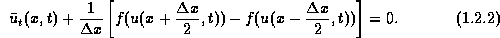Next, we introduce a small time-step,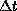, and integrate over the slab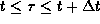,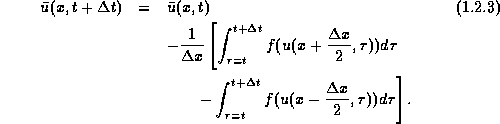We end up with an equivalent reformulation of the conservation law (1.2.1): it expresses the precise relation between the sliding averages,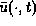, and their underlying pointvalues,. We shall use this reformulation, (1.2.3), as the starting point for the construction of Godunov-type schemes.

We construct an approximate solution,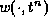, at the discrete time-levels,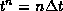. Here,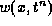is a piecewise polynomial written in the form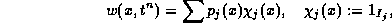where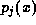are algebraic polynomials supported at the discrete cells,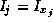, centered around the midpoints,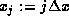. An exact evolution ofbased on (1.2.3), reads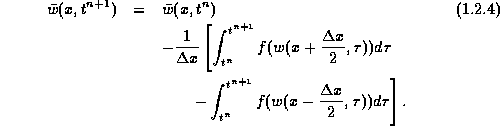To construct a Godunov-type scheme, we realize (1.2.4) -- or at least an accurate approximation of it, at discrete gridpoints. Here, we distinguish between the main methods, according to their way of sampling (1.2.4): these two main sampling methods correspond to upwind schemes and central schemes.